# Hill Cipher | Cyber Security

In this article, we will learn what Hill Cipher is? It's implementation technique: How to encrypt and decrypt strings using Hill Cipher and finally, we will encrypt and decrypt a string using this technique.
Submitted by Sahil Singh, on September 28, 2019

Now, Hill Cipher is a very basic cryptographic technique which is used to convert a string into ciphertext. This technique was invented by an American Mathematician "Lester Sanders Hill". This is a polygraphic substitution cipher because the substitution or encryption is performed on the block on letters. It works on multiple letters at the same time. It was the first polygraphic cipher which was operable on more than 3 symbols.

Implementation Technique

### Encryption

In Hill Cipher, each letter is represented by a number modulo 26. So according to this, A = 0, B = 1, C = 2, ..., Z = 25. A string will be given to you and you have to make a column matrix using the letter-numberer relationship shown above. We'll be given a key matrix as well. We must ensure that | Key | should not be zero otherwise we can not apply hill cipher technique. This key matrix will be used in decryption as well. We need to multiply these two matrices. The formula for the ciphertext using Hill Cipher is: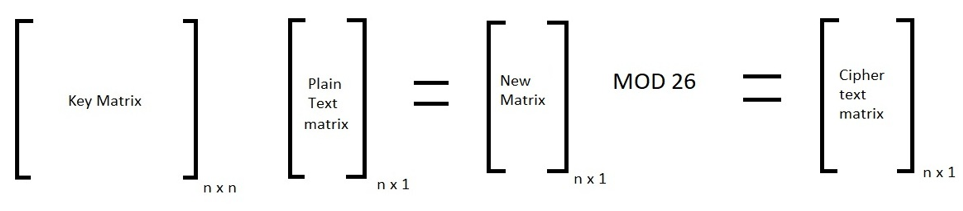Here, n is the order of matrix or we can say the n is the number of letters we are taking at once to encrypt. Now, you'll get column matrix of order n x 1 which will have numbers from 0 to 25. You just have to replace the number with the letters from the relationship shown above and your ciphertext is ready.

### Decryption

The letter-numberer relationship will remain the same in the decryption part. We'll use the inverse of the key matrix in decryption. Order of the matrix will remain the same. We need to multiply the inverse of the key matrix with the cipher text matrix we've got from the encryption technique. The formula is given below: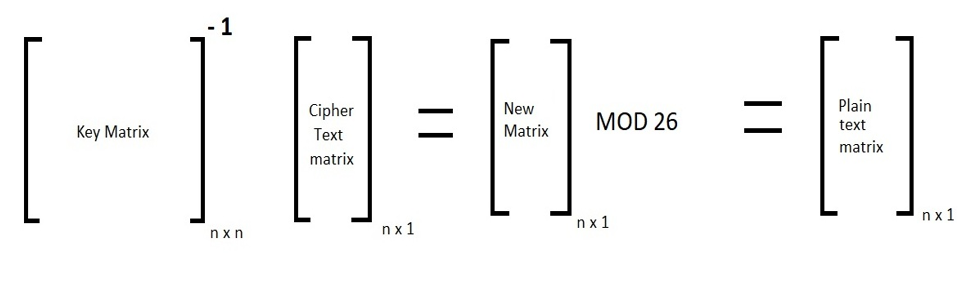Example:

Let us take plain text: DOG and the key matrix is: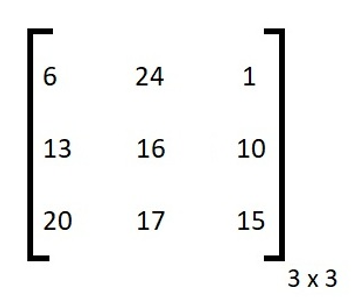D = 3, O = 14 , G = 6 so our column matrix is: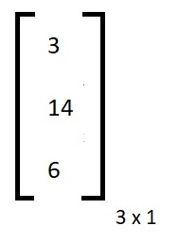Now we have to apply the multiplication as shown in the encryption formula. After multiplying and applying modulo 26 we got this cipher text matrix: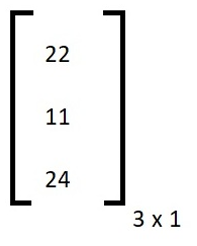Now, 22 = W, 11 = L, 24 = Y

So cipher text of DOG is WLY

Now we'll do decryption and we'll use the generated ciphertext to convert it to plain text. First of all, we need to find the inverse of the key matrix. So the inverse of our key matrix is: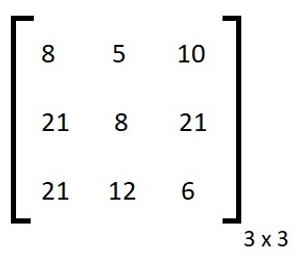Now after multiplying the inverse matrix with the ciphertext matrix that we've generated in the encryption, we can get out plain text matrix again.

So after multiplying and taking modulo 26, the decrypted matrix is: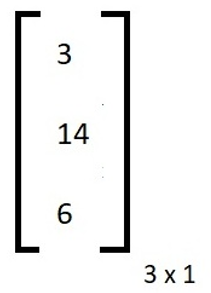3 = D, 14 = 0 and 6 = G, So this is equal to the plain text it means our encryption and decryption both are right. Now, the Hill Cipher technique is over.